##Strength calculation of structures (APM StructFEM)

The Struct the APM to FEM is designed to solve a wide range of engineering problems. The system is based on the mathematical kernel of finite element analysis of the APM the Structure 3, the D .

## The composition of APM StructFEMAPM Structure3D - the module for calculating the stress-strain state, stability, own and forced oscillations of parts and structures by the finite element methodAPM Graph - a flat parametric drawing and graphics editor with a tool for calculating dimensional chainsAPM Studio - pre- and postprocessor for creating models for strength (finite element analysis) with the ability to import STEP filesAPM Joint - module for calculating and designing connections of machine elementsAPM Base - module for creating and editing databasesAPM Section Data - database of parametric sectionsAPM Mechanical Data - database of graphic information of standard parts and assemblies, reference data on engineeringAPM Material Data - material parameters databaseAPM Construction Data - database of graphic information on standard parts and elements of building structures

## Functionality APM StructFEM

Linear solutions

• Calculation of stress-strain state (static calculation)
• Calculation of safety factors and buckling

Nonlinear solutions

• Calculation of stress-strain state in view of the geometric and physical nonlinearity
• Calculation of stress-strain state in the case of contact interaction
• Calculation in the case of very large-scale deformation in view of geometrical and physical nonlinearity.
• Modeling of shock interaction (crash tests)

dynamic analysis

• Determination of frequencies and modes of vibration including
• Calculation of the forced vibrations modeling system responses
real-time mode with a given law of variation of the driving load
• Calculation of fatigue strength under the influence of cyclical external influence at a constant, variable and random modes loaded
• Calculation of vibration bases
• Modeling the behavior of structures under seismic actions

Types of finite elements (to create a computational model)

• Rod arbitrary cross-sections
• Flexible elements sided hardness: ropes, cables and shrouds, etc.
• The shell and plate: isoparametric first order 3,
4 and the second 6 and node 8
• Solid volume: isoparametric first order (4, 8)
• Tubular
• Special elements: elastic connection, elastic supports, contact elements, concentrated masses and moments of inertia, etc.
• Superelement method substructures

Types of materials

• Isotropic
• orthotropic
• Anisotropic
• Multilayer
• Composite

The boundary conditions allow for the imposition of restrictions through the use of:

• absolutely rigid supports and pillars with partly exempt bonds
• elastic supports bi-directional communications
• elastic supports with unidirectional links
• rigid connections of elements, compounds with partially exempt bonds and connections eccentric elements
• supports with the given values ​​of linear and angular displacements possible
• contact elements

• Concentrated forces and moments (fixed and variable over time)
• Distributed loads along the length, area and volume of the load (constant, variable depending on the coordinates and time-varying)
• Loads specified linear and / or angular displacement (constant
and time-varying)
• Snow, wind (taking into account the fluctuating wind component),
as well as seismic loads (SNIP), taking into account the distributed
and concentrated masses, linear and rotational degrees of freedom
• Hydrostatic pressure type
• The pressure contact type
• The calculated combination of effort (DCS)
• Centrifugal (defined linear and / or angular acceleration)
• gravity

The results of the calculations are as follows:

• Maps and diagrams of equivalent stresses and their components and the principal stresses
• Maps and diagrams of linear, angular and total displacements
• Maps and diagrams of distribution of deformations in design elements
• Maps and diagrams of internal force distribution
• The map of distribution efforts in the contact zone
• Safety factors and buckling
• map allocation of safety factors and the number of the criterion of fatigue strength cycles
• Map allocation of safety factors for strength and durability
• Map of the distribution of the accumulated per unit volume of the internal strain energy
• coordinates of the center of gravity, weight, volume, length, surface area, moments of inertia design model
• Moments of inertia, static moments and cross-sectional areas
• The bearing forces and total reaction model shown in the center of gravity
• An animated presentation of the results

distribution maps are available both on the surface and the rod section and the volume elements.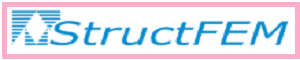APM STRUCT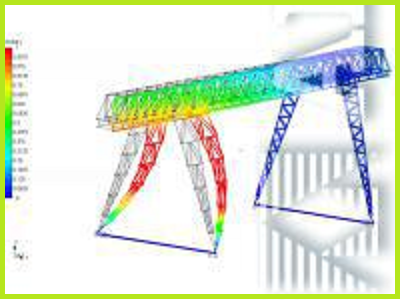3D STRUCT 01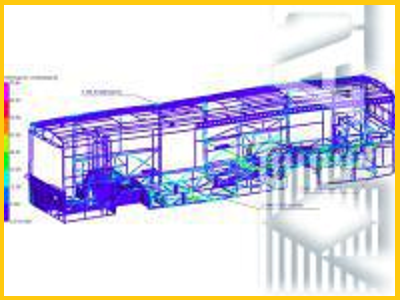3D STRUCT 02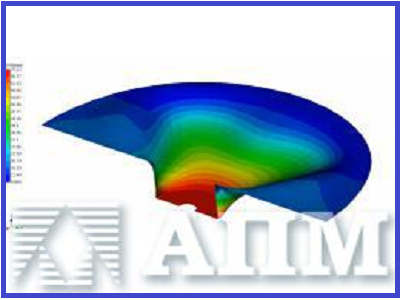3D STRUCT 03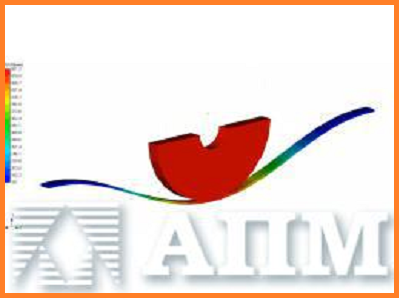3D STRUCT 04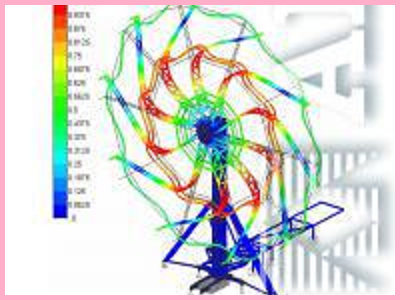3D STRUCT 05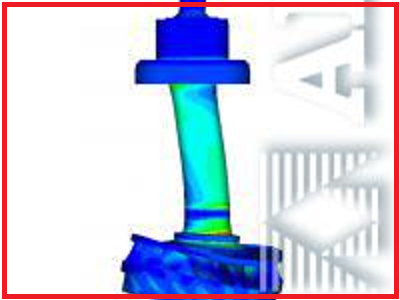3D STRUCT 06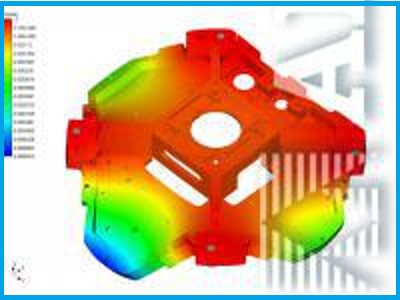3D STRUCT 07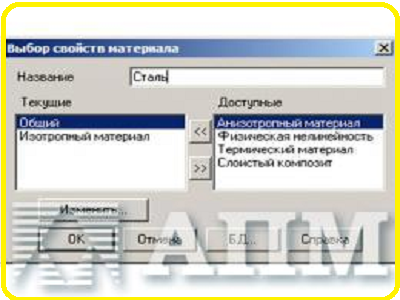3D STRUCT 08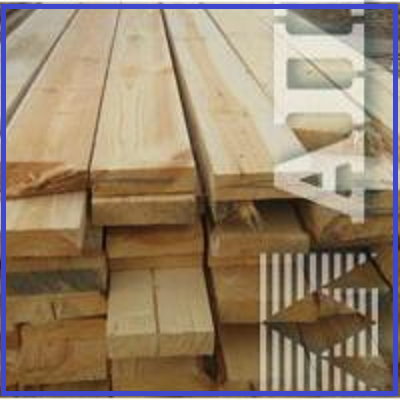3D STRUCT 09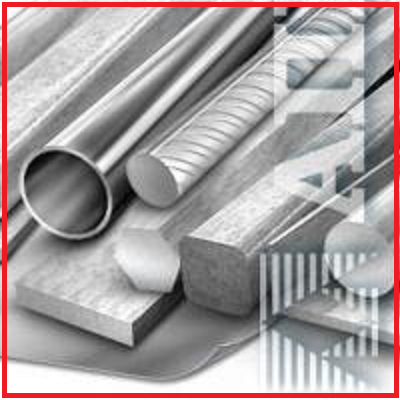3D STRUCT 10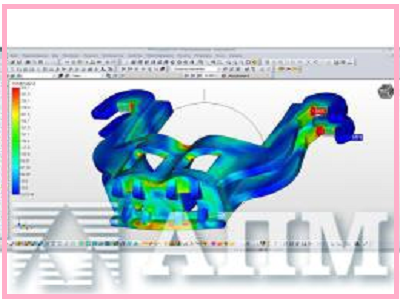3D STRUCT 17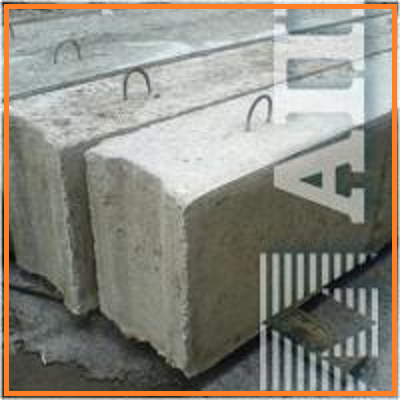3D STRUCT 11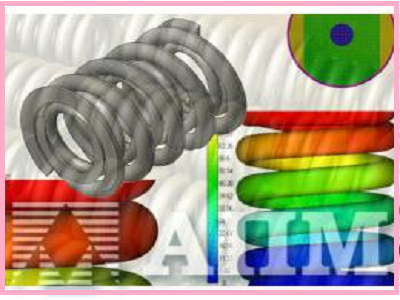3D STRUCT 12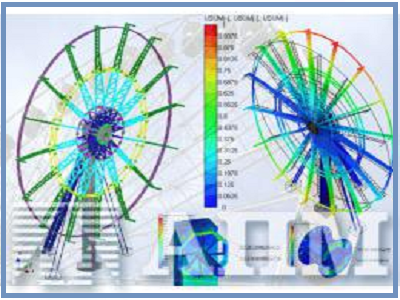3D STRUCT 13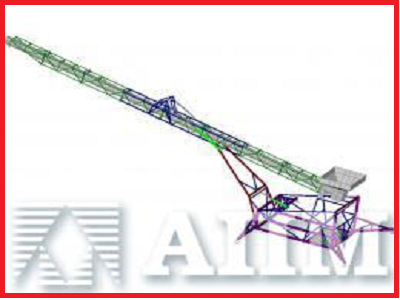3D STRUCT 14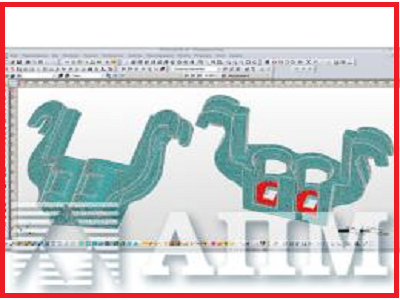3D STRUCT 15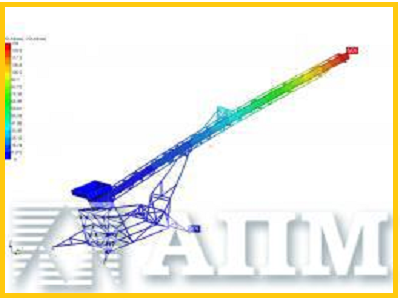3D STRUCT 16
info@paviathintegratedsolution.com www.paviathintegratedsolution.com